Question

# Question 2 (20 points): Consider the functions f(x, y)-xe y sin y and g(x, y)-ys 1. Show f is differentiable in its domain 2. Compute the partial derivatives of g at (0,0) 3. Show that g is not d...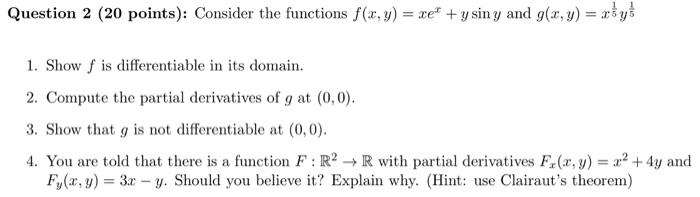Question 2 (20 points): Consider the functions f(x, y)-xe y sin y and g(x, y)-ys 1. Show f is differentiable in its domain 2. Compute the partial derivatives of g at (0,0) 3. Show that g is not differentiable at (0,0) 4. You are told that there is a function F : R2 → R with partial derivatives F(x,y) = x2 +4y and Fy(x, y 3x - y. Should you believe it? Explain why. (Hint: use Clairaut's theorem)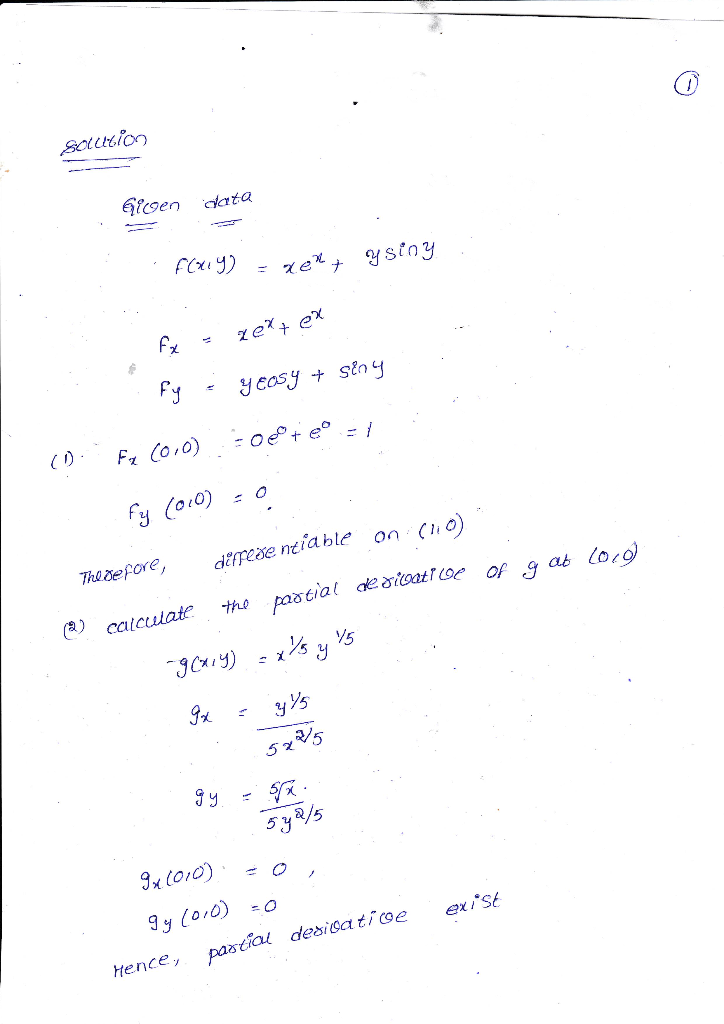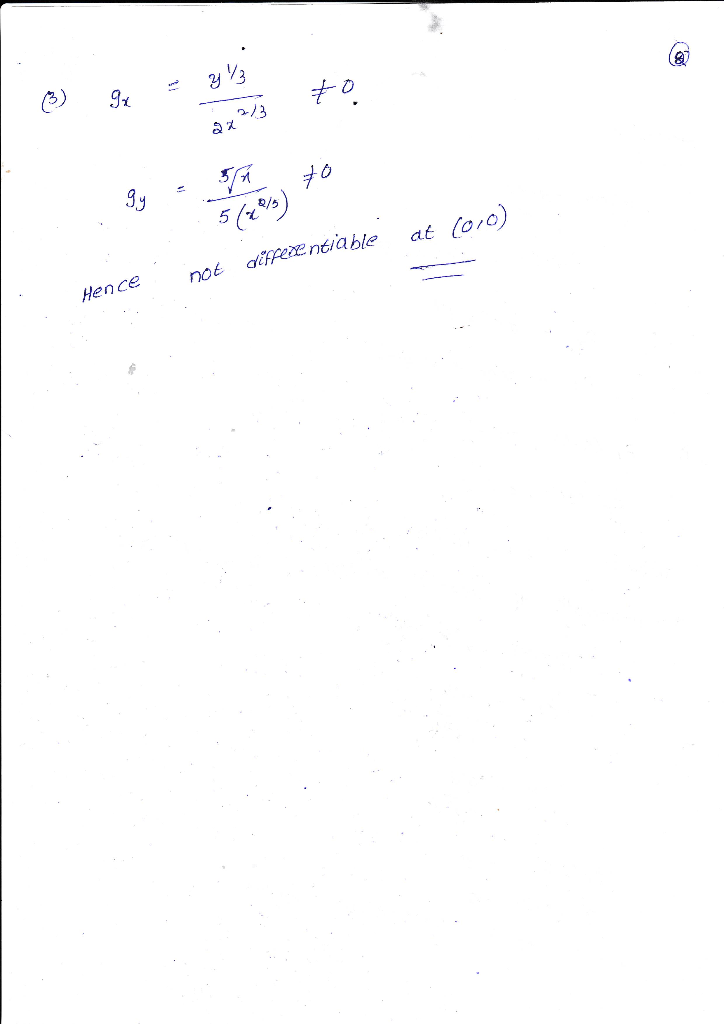#### Earn Coins

Coins can be redeemed for fabulous gifts.

Similar Homework Help Questions
• ### showing multivariable calculus functions are differentiable Please help! 2. Recall that by Theorem 3 of Section 14.3, a...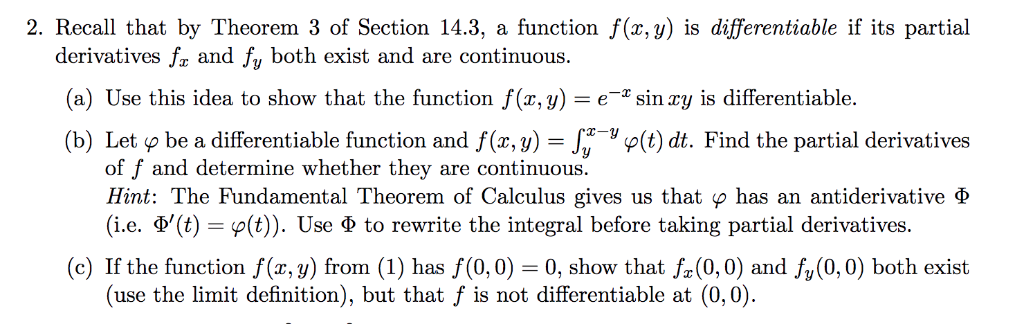showing multivariable calculus functions are differentiable Please help! 2. Recall that by Theorem 3 of Section 14.3, a function f(x,y) is differentiable if its partial derivatives fa and fy both exist and are continuous. (a) Use this idea to show that the function f(x,y)-esin ry is differentiable. (b) Let o be a differentiable function and f(,)Jy Find the partial derivatives of f and determine whether they are continuous. Hint: The Fundamental Theorem of Calculus gives us that Ø has an...

• ### Problem 3. Define the function: 2+_ 0 if (z,y)#10.0) if (a,y)-(0,0) f(x, v)= (a) Graph the...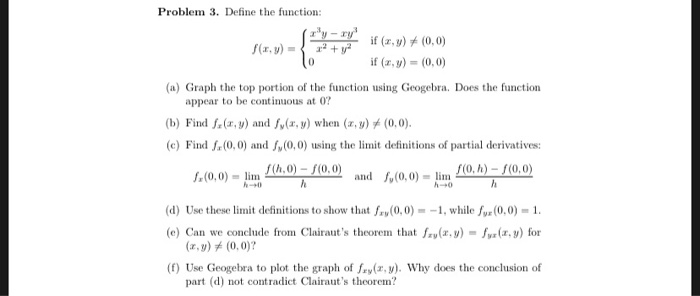Problem 3. Define the function: 2+_ 0 if (z,y)#10.0) if (a,y)-(0,0) f(x, v)= (a) Graph the top portion of the function using Geogebra. Does the function appear to be continuus at 0? (b) Find fz(z, y) and fy(z, y) when (z, y) #10.0) (c) Find f(0,0) and s,(0,0) using the limit definitions of partial derivatives and f,(0,0)-lim rah) - f(O,0) d) Use these limit definitions to show that fay(0,0)--1, while x(0,0)-1 (e) Can we conclude from Clairaut's theorem that()-yr(x,y) for...

• ### 4. Consider the functions f : R2 R2 and g R2 R2 given by f(x, y) (x, xy) and g(x, y)-(x2 + y, x +...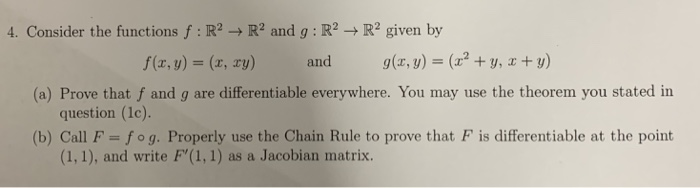4. Consider the functions f : R2 R2 and g R2 R2 given by f(x, y) (x, xy) and g(x, y)-(x2 + y, x + y) (a) Prove that f and g are differentiable everywhere. You may use the theorem you stated in (b) Call F-fog. Properly use the Chain Rule to prove that F is differentiable at the point question (1c). (1,1), and write F'(1, 1) as a Jacobian matrix. 4. Consider the functions f : R2 R2 and...

• ### GROUP WORK 1, SECTION 14.3 Clarifying Clairaut's Theorem Consider f (x, y, z) = x?cos (y...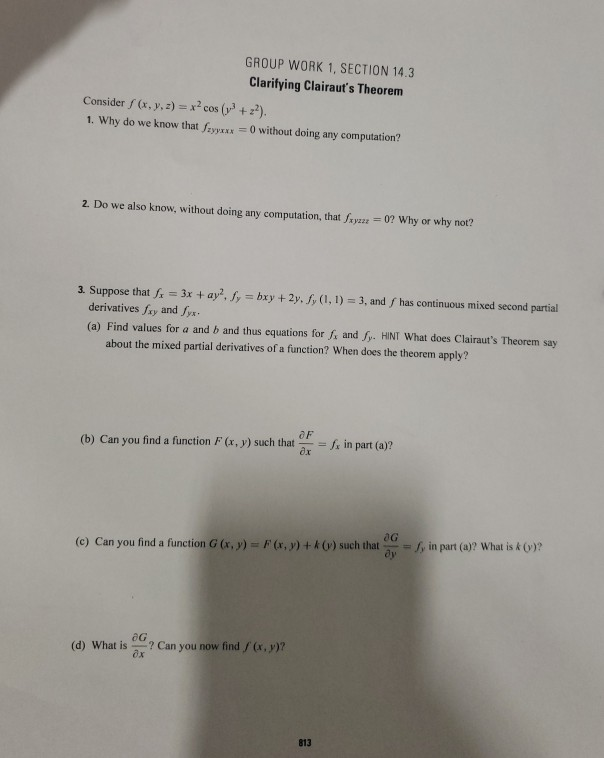GROUP WORK 1, SECTION 14.3 Clarifying Clairaut's Theorem Consider f (x, y, z) = x?cos (y + 2). 1. Why do we know that fyyxxx=0 without doing any computation? 2. Do we also know, without doing any computation, that Sxyz = 0? Why or why not? 3. Suppose that a = 3x + ay". Jy = bxy + 2y. S,(1, 1) = 3, and has continuous mixed second partial derivatives xy and fyx. (a) Find values for a and b...

• ### 2. Consider the function f : R2 → R defined below. r3уг_ if (x,y) (0,0) f(x,y)...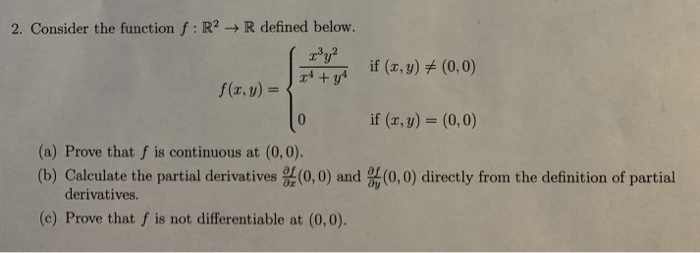2. Consider the function f : R2 → R defined below. r3уг_ if (x,y) (0,0) f(x,y) = if (x, y) (0, 0) (a) Prove that f is continuous at (0,0) (b) Calculate the partial derivatives (0,0) and (0,0) directly from the definition of partial derivatives. (c) Prove that f is not differentiable at (0,0).

• ### 3. (7 points) Consider the function sin f (x, y) = { if (x, y) +...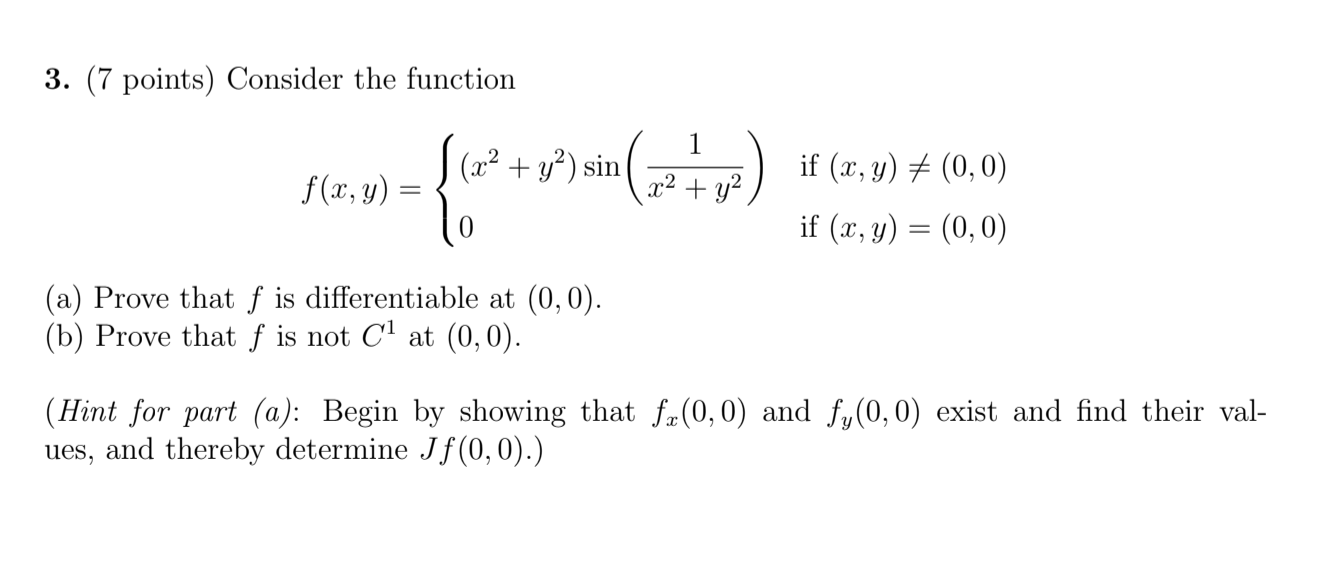3. (7 points) Consider the function sin f (x, y) = { if (x, y) + (0,0) if (x, y) = (0,0) (a) Prove that f is differentiable at (0,0). (b) Prove that f is not C1 at (0,0). (Hint for part (a): Begin by showing that fx(0,0) and fy(0,0) exist and find their val- ues, and thereby determine Jf(0,0).)

• ### Let f(x,y) = (x" + 2?y?)!. compute all second-order partial derivatives of fat (0,0), if they...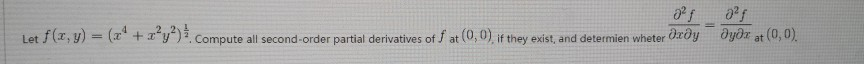Let f(x,y) = (x" + 2?y?)!. compute all second-order partial derivatives of fat (0,0), if they exist, and determien wheter dæðyəyər at (0,0).

• ### if (r.y) (0,0), 0,f (, y) (0, 0) 2. Consider f : IR2 -R defined by f(r,y)-+ (a) Show by explicit computation that the directional derivative exists at (x, y)- (0,0) for all oi rections u є R2 with 1...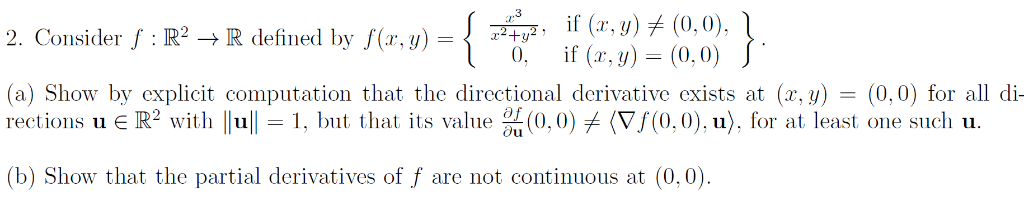if (r.y) (0,0), 0,f (, y) (0, 0) 2. Consider f : IR2 -R defined by f(r,y)-+ (a) Show by explicit computation that the directional derivative exists at (x, y)- (0,0) for all oi rections u є R2 with 1 11-1, but that its value %(0.0) (Vf(0,0).u), fr at least one sucli u. (b) Show that the partial derivatives of f are not continuous at (0,0) if (r.y) (0,0), 0,f (, y) (0, 0) 2. Consider f : IR2 -R...

• ### 1. (20 points) Find derivatives of the following functions. (a) f(x) = 1012 (b) g(x) =...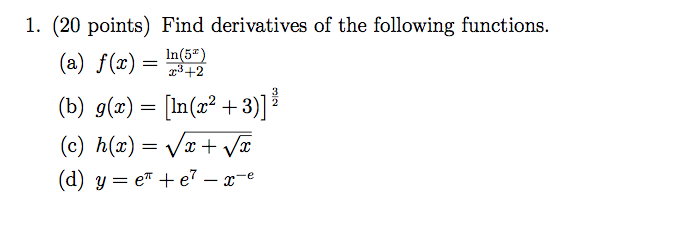1. (20 points) Find derivatives of the following functions. (a) f(x) = 1012 (b) g(x) = (ln(x2 + 3)] (c) h(x) = Vx+V2 (d) y=et +e? – x-e

• ### (1 point) Consider the function defined by F(x, y) = x2 + y2 except at (r, y) - (0, 0) where F(0,0)0 Then we have (0,0)...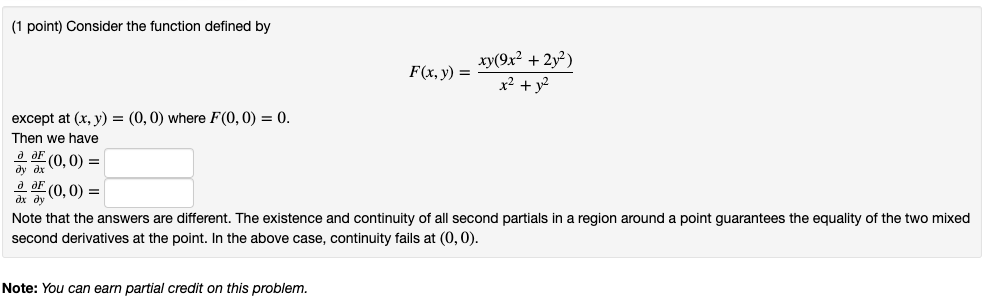(1 point) Consider the function defined by F(x, y) = x2 + y2 except at (r, y) - (0, 0) where F(0,0)0 Then we have (0,0) = (0,0) = ax dy Note that the answers are different. The existence and continuity of all second partials in a region around a point guarantees the equality of the two mixed second derivatives at the point. In the above case, continuity fails at (0,0) Note: You can earn partial credit on this problem...